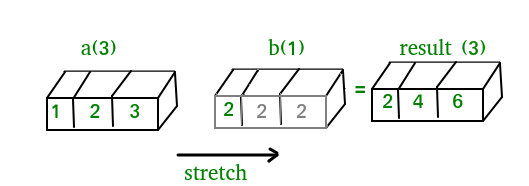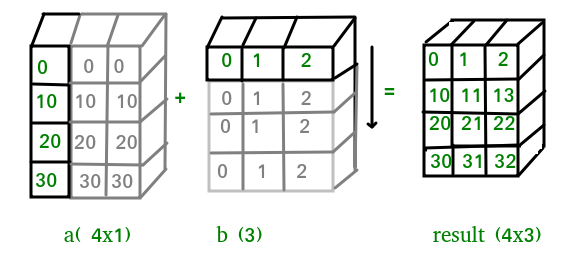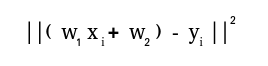Open in App
Not now

# NumPy in Python | Set 2 (Advanced)

• Difficulty Level : Medium
• Last Updated : 09 Feb, 2018

NumPy in Python | Set 1 (Introduction)

1. Stacking: Several arrays can be stacked together along different axes.
• np.vstack: To stack arrays along vertical axis.
• np.hstack: To stack arrays along horizontal axis.
• np.column_stack: To stack 1-D arrays as columns into 2-D arrays.
• np.concatenate: To stack arrays along specified axis (axis is passed as argument).

 `import` `numpy as np ` ` `  `a ``=` `np.array([[``1``, ``2``], ` `              ``[``3``, ``4``]]) ` ` `  `b ``=` `np.array([[``5``, ``6``], ` `              ``[``7``, ``8``]]) ` ` `  `# vertical stacking ` `print``(``"Vertical stacking:\n"``, np.vstack((a, b))) ` ` `  `# horizontal stacking ` `print``(``"\nHorizontal stacking:\n"``, np.hstack((a, b))) ` ` `  `c ``=` `[``5``, ``6``] ` ` `  `# stacking columns ` `print``(``"\nColumn stacking:\n"``, np.column_stack((a, c))) ` ` `  `# concatenation method  ` `print``(``"\nConcatenating to 2nd axis:\n"``, np.concatenate((a, b), ``1``)) `

Output:

```Vertical stacking:
[[1 2]
[3 4]
[5 6]
[7 8]]

Horizontal stacking:
[[1 2 5 6]
[3 4 7 8]]

Column stacking:
[[1 2 5]
[3 4 6]]

Concatenating to 2nd axis:
[[1 2 5 6]
[3 4 7 8]]```
2. Splitting:For splitting, we have these functions:
• np.hsplit: Split array along horizontal axis.
• np.vsplit: Split array along vertical axis.
• np.array_split: Split array along specified axis.

 `import` `numpy as np ` ` `  `a ``=` `np.array([[``1``, ``3``, ``5``, ``7``, ``9``, ``11``], ` `              ``[``2``, ``4``, ``6``, ``8``, ``10``, ``12``]]) ` ` `  `# horizontal splitting ` `print``(``"Splitting along horizontal axis into 2 parts:\n"``, np.hsplit(a, ``2``)) ` ` `  `# vertical splitting ` `print``(``"\nSplitting along vertical axis into 2 parts:\n"``, np.vsplit(a, ``2``))`

Output:

```Splitting along horizontal axis into 2 parts:
[array([[1, 3, 5],
[2, 4, 6]]), array([[ 7,  9, 11],
[ 8, 10, 12]])]

Splitting along vertical axis into 2 parts:
[array([[ 1,  3,  5,  7,  9, 11]]), array([[ 2,  4,  6,  8, 10, 12]])]```
3. Broadcasting: The term broadcasting describes how NumPy treats arrays with different shapes during arithmetic operations. Subject to certain constraints, the smaller array is “broadcast” across the larger array so that they have compatible shapes.

Broadcasting provides a means of vectorizing array operations so that looping occurs in C instead of Python. It does this without making needless copies of data and usually leads to efficient algorithm implementations. There are also cases where broadcasting is a bad idea because it leads to inefficient use of memory that slows computation.

NumPy operations are usually done element-by-element which requires two arrays to have exactly the same shape. Numpy’s broadcasting rule relaxes this constraint when the arrays’ shapes meet certain constraints.

The Broadcasting Rule: In order to broadcast, the size of the trailing axes for both arrays in an operation must either be the same size or one of them must be one.

Let us see some examples:

``````A(2-D array): 4 x 3
B(1-D array):     3
Result      : 4 x 3
``````
``````A(4-D array): 7 x 1 x 6 x 1
B(3-D array):     3 x 1 x 5
Result      : 7 x 3 x 6 x 5
``````

But this would be a mismatch:

``````A: 4 x 3
B:     4
``````

The simplest broadcasting example occurs when an array and a scalar value are combined in an operation.
Consider the example given below:

 `import` `numpy as np ` ` `  `a ``=` `np.array([``1.0``, ``2.0``, ``3.0``]) ` ` `  `# Example 1 ` `b ``=` `2.0` `print``(a ``*` `b) ` ` `  `# Example 2 ` `c ``=` `[``2.0``, ``2.0``, ``2.0``] ` `print``(a ``*` `c)`

Output:

```[ 2.  4.  6.]
[ 2.  4.  6.]```

We can think of the scalar b being stretched during the arithmetic operation into an array with the same shape as a. The new elements in b, as shown in above figure, are simply copies of the original scalar. Although, the stretching analogy is only conceptual.
Numpy is smart enough to use the original scalar value without actually making copies so that broadcasting operations are as memory and computationally efficient as possible. Because Example 1 moves less memory, (b is a scalar, not an array) around during the multiplication, it is about 10% faster than Example 2 using the standard numpy on Windows 2000 with one million element arrays!
The figure below makes the concept more clear:In above example, the scalar b is stretched to become an array of with the same shape as a so the shapes are compatible for element-by-element multiplication.

Now, let us see an example where both arrays get stretched.

 `import` `numpy as np ` ` `  `a ``=` `np.array([``0.0``, ``10.0``, ``20.0``, ``30.0``]) ` `b ``=` `np.array([``0.0``, ``1.0``, ``2.0``]) ` ` `  `print``(a[:, np.newaxis] ``+` `b)`

Output:

```[[  0.   1.   2.]
[ 10.  11.  12.]
[ 20.  21.  22.]
[ 30.  31.  32.]]
```In some cases, broadcasting stretches both arrays to form an output array larger than either of the initial arrays.

4. Working with datetime: Numpy has core array data types which natively support datetime functionality. The data type is called “datetime64”, so named because “datetime” is already taken by the datetime library included in Python.
Consider the example below for some examples:

 `import` `numpy as np ` ` `  `# creating a date ` `today ``=` `np.datetime64(``'2017-02-12'``) ` `print``(``"Date is:"``, today) ` `print``(``"Year is:"``, np.datetime64(today, ``'Y'``)) ` ` `  `# creating array of dates in a month ` `dates ``=` `np.arange(``'2017-02'``, ``'2017-03'``, dtype``=``'datetime64[D]'``) ` `print``(``"\nDates of February, 2017:\n"``, dates) ` `print``(``"Today is February:"``, today ``in` `dates) ` ` `  `# arithmetic operation on dates ` `dur ``=` `np.datetime64(``'2017-05-22'``) ``-` `np.datetime64(``'2016-05-22'``) ` `print``(``"\nNo. of days:"``, dur) ` `print``(``"No. of weeks:"``, np.timedelta64(dur, ``'W'``)) ` ` `  `# sorting dates ` `a ``=` `np.array([``'2017-02-12'``, ``'2016-10-13'``, ``'2019-05-22'``], dtype``=``'datetime64'``) ` `print``(``"\nDates in sorted order:"``, np.sort(a))`

Output:

```Date is: 2017-02-12
Year is: 2017

Dates of February, 2017:
['2017-02-01' '2017-02-02' '2017-02-03' '2017-02-04' '2017-02-05'
'2017-02-06' '2017-02-07' '2017-02-08' '2017-02-09' '2017-02-10'
'2017-02-11' '2017-02-12' '2017-02-13' '2017-02-14' '2017-02-15'
'2017-02-16' '2017-02-17' '2017-02-18' '2017-02-19' '2017-02-20'
'2017-02-21' '2017-02-22' '2017-02-23' '2017-02-24' '2017-02-25'
'2017-02-26' '2017-02-27' '2017-02-28']
Today is February: True

No. of days: 365 days
No. of weeks: 52 weeks

Dates in sorted order: ['2016-10-13' '2017-02-12' '2019-05-22']```
5. Linear algebra in NumPy: The Linear Algebra module of NumPy offers various methods to apply linear algebra on any numpy array.
You can find:

• rank, determinant, trace, etc. of an array.
• eigen values of matrices
• matrix and vector products (dot, inner, outer,etc. product), matrix exponentiation
• solve linear or tensor equations and much more!

Consider the example below which explains how we can use NumPy to do some matrix operations.

 `import` `numpy as np ` ` `  `A ``=` `np.array([[``6``, ``1``, ``1``], ` `              ``[``4``, ``-``2``, ``5``], ` `              ``[``2``, ``8``, ``7``]]) ` ` `  `print``(``"Rank of A:"``, np.linalg.matrix_rank(A)) ` ` `  `print``(``"\nTrace of A:"``, np.trace(A)) ` ` `  `print``(``"\nDeterminant of A:"``, np.linalg.det(A)) ` ` `  `print``(``"\nInverse of A:\n"``, np.linalg.inv(A)) ` ` `  `print``(``"\nMatrix A raised to power 3:\n"``, np.linalg.matrix_power(A, ``3``))`

Output:

```Rank of A: 3

Trace of A: 11

Determinant of A: -306.0

Inverse of A:
[[ 0.17647059 -0.00326797 -0.02287582]
[ 0.05882353 -0.13071895  0.08496732]
[-0.11764706  0.1503268   0.05228758]]

Matrix A raised to power 3:
[[336 162 228]
[406 162 469]
[698 702 905]]```

Let us assume that we want to solve this linear equation set:

``````x + 2*y = 8
3*x + 4*y = 18
``````

This problem can be solved using linalg.solve method as shown in example below:

 `import` `numpy as np ` ` `  `# coefficients ` `a ``=` `np.array([[``1``, ``2``], [``3``, ``4``]]) ` `# constants ` `b ``=` `np.array([``8``, ``18``]) ` ` `  `print``(``"Solution of linear equations:"``, np.linalg.solve(a, b))`

Output:

`Solution of linear equations: [ 2.  3.]`

Finally, we see an example which shows how one can perform linear regression using least squares method.

A linear regression line is of the form w1x + w2 = y and it is the line that minimizes the sum of the squares of the distance from each data point to the line. So, given n pairs of data (xi, yi), the parameters that we are looking for are w1 and w2 which minimize the error:Let us have a look at the example below:

 `import` `numpy as np ` `import` `matplotlib.pyplot as plt ` ` `  `# x co-ordinates ` `x ``=` `np.arange(``0``, ``9``) ` `A ``=` `np.array([x, np.ones(``9``)]) ` ` `  `# linearly generated sequence ` `y ``=` `[``19``, ``20``, ``20.5``, ``21.5``, ``22``, ``23``, ``23``, ``25.5``, ``24``] ` `# obtaining the parameters of regression line ` `w ``=` `np.linalg.lstsq(A.T, y)[``0``]  ` ` `  `# plotting the line ` `line ``=` `w[``0``]``*``x ``+` `w[``1``] ``# regression line ` `plt.plot(x, line, ``'r-'``) ` `plt.plot(x, y, ``'o'``) ` `plt.show()`

Output:So, this leads to the conclusion of this series of NumPy tutorial.

NumPy is a widely used general purpose library which is at the core of many other computation libraries like scipy, scikit-learn, tensorflow, matplotlib, opencv, etc. Having a basic understanding of NumPy helps in dealing with other higher level libraries efficiently!

References:

My Personal Notes arrow_drop_up
Related Articles